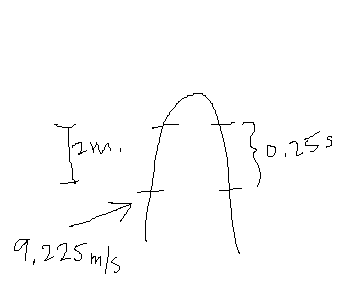# Free-fall problem

PhizKid

## Homework Statement

A ball thrown up falls back down in front of a window from where an observer sees the ball for a total of 0.5 seconds. The window is 2 m. tall. What is the maximum height of the ball?

## Homework Equations

1D Kinematic equations

## The Attempt at a SolutionI got that initial velocity from the bottom of the window by using $$V^2 = V_0^2 + 2a(x - x_0)$$.

I set the seconds to 0.25 since 0.5 is the total, and -9.8 for the acceleration of gravity.

Then I plugged all of that back into $$V^2 = V_0^2 + 2a(x - x_0)$$ by setting V^2 = 0 because the velocity at the highest point is 0, V_0 is the previously found initial velocity, accleration is -9.8 again, and x - x_0 is the unknown. I got 1.88 m., which is obviously wrong because the window itself is 2m. But I guess it could be the answer, but I'm not sure. It doesn't look right.

I got that initial velocity from the bottom of the window by using $$V^2 = V_0^2 + 2a(x - x_0)$$.